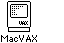## Comment

#<expr>[Rx] The operand is at the address (Rx * operand size) + PC.
(Assembles to (PC)+[Rx]. This is NOT a useful mode)
<expr>[Rx] The operand is at the address (Rx * operand size)+<expr>.
(PC relative. Assembles to <expr> - current_location (PC) [Rx] ).
@#<expr>[Rx] The operand is at the address (Rx * operand size)+<expr>.
(This has the same effect as <expr>[Rx] but assembles to @(PC)+[Rx]).
@<expr>[Rx] The operand is at the address (Rx * operand size) + the value (long) at the address <expr> .
(Compiles to @<expr> - current_location(PC)[Rx] ).
(Rn)[Rx] The operand is at the address (Rx * operand size) + Rn
-(Rn)[Rx] The value of Rn is decremented by the size of the operand type before adding it to (Rx * operand size) to obtain the address of the operand.
(Rn)+[Rx] The value of Rn is incremented by the size of the operand type after adding it to (Rx * operand size) to obtain the address of the operand.
@(Rn)+[Rx] The operands address is obtained by taking the value at the memory address specified by the contents of Rn and adding it to (Rx * operand size) . The contents of Rn are then incremented by 4.
<expr>(Rn)[Rx] The address of the operand is obtained by
<expr> + Rn + (Rx * operand size) .
@<expr>(Rn)[Rx] The operands address is obtained by taking the value at the address (<expr> + Rn) and adding it to (Rx * operand size) .
Previous Page

### Notes

• <expr> is an expression as described (see XXX).
• An <expr> is evaluated at assembly time.
• Rn and Rx are any registers and may be the same register (be careful if they you do this).
• The assembler recognises the UNIX;
"*" as equivalent to the VMS "@"
"\$" as equivalent to the VMS "#",
But MacVAX will always display these in the VMS form used above.